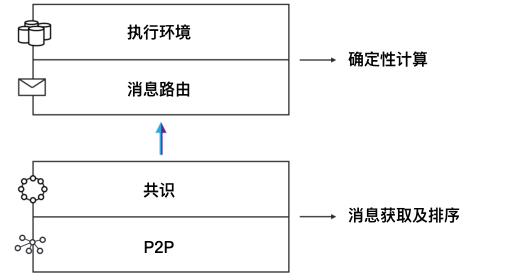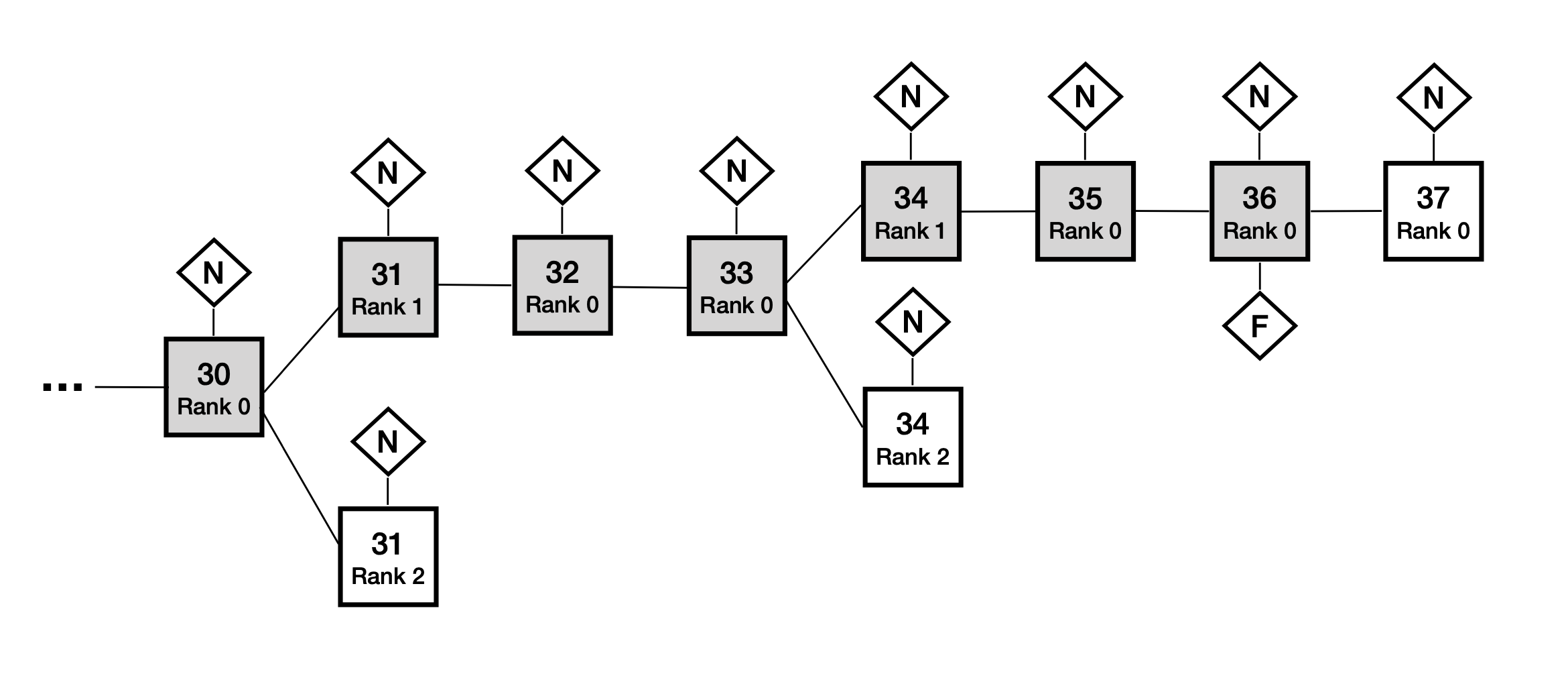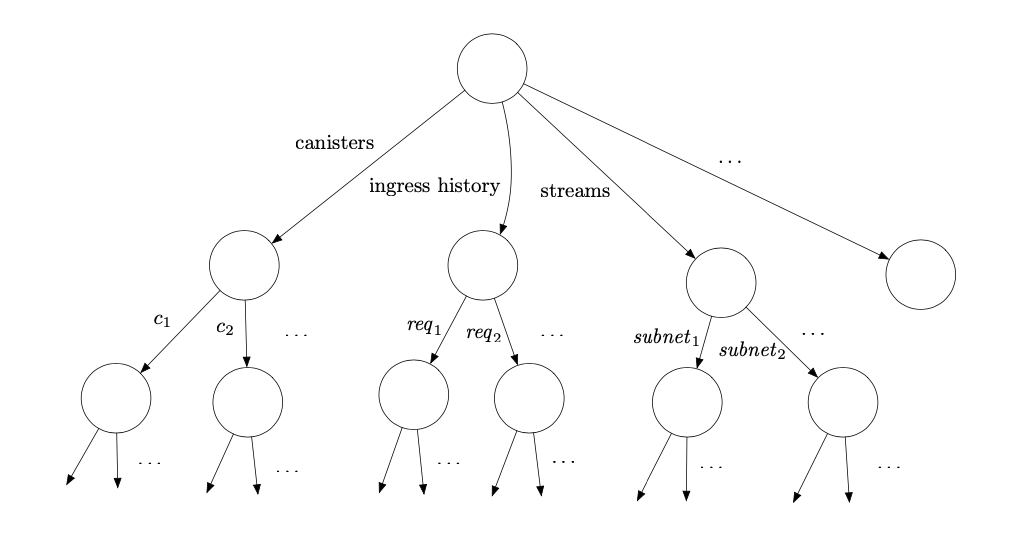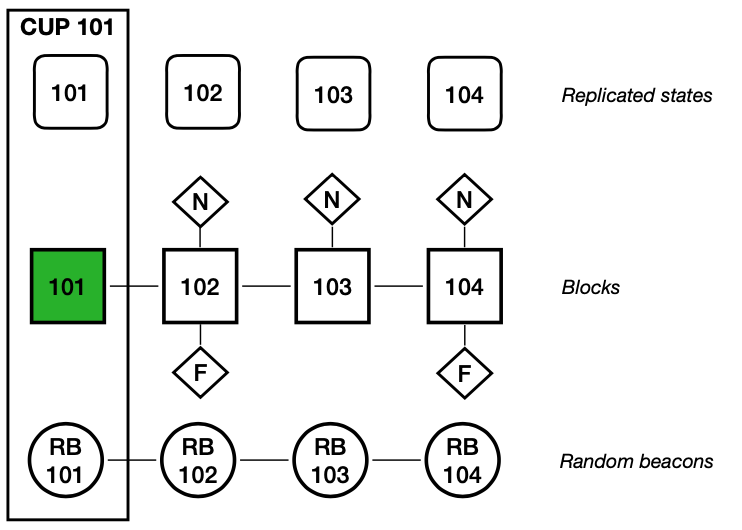# 白皮书

——The Internet Computer for Geeks(v1.3)
Dfinity团队
2022年4月19日

Github Repo

## 1 引言​

### 1.1 释放智能合约​

IC是一个新的运行智能合约的平台。在这里，我们使用术语“智能合约”广义上的定义：一种通用的防篡改的计算机程序，其在分布式公共网络自治地执行。

• 通用的，是指智能合约这类程序是图灵完备的（即任何可计算的运算都可以用智能合约完成）。
• 防篡改的，是指程序的指令被可信地执行，并且计算的中间结果和最终结果被准确地存储和/或传输。
• 自治地，是指智能合约被网络自动执行，不需要任何人采取任何行动。
• 分布式公共网络，是指计算机网络可被公开地访问的、地理上分布式的，并且不受少数人或组织控制。

• 可组合的，意味着他们可以彼此交互。
• 支持代币化，意味着他们可以使用和交易代币。

• 更具成本优势，特别是允许应用程序以先前平台的一小部分成本，来计算和存储数据；
• 为智能合约的交易处理提供更高的吞吐量更低的延迟
• 更具扩展性，特别是IC可以原生地处理无限量的智能合约数据和计算，因为它可以通过向网络中添加节点来提升容量。

• 提供互操作性，共享函数，持久化APIs和无主应用程序，上述的所有特点减少了平台的风险，并鼓励创新和协作。
• 自动持久化数据于内存中，无需数据库服务器和存储管理，提升了计算效率并简化了软件开发。
• 简化了IT组织需要集成和管理的技术栈，提升了运营效率。

### 1.2 高阶视角下的IC​

IC中的状态转换函数是一个通用函数，意味着一些存储在状态中的输入和数据可能是任意的程序，这些程序会作用于其他的输入和数据。因此，这样的一个状态机代表了一个通用（即图灵完备）的计算模型。

### 1.4 通信模型​

• 同步模型中，存在已知的有限时间限制 $\delta$ ，因此对于发送的任意消息，它会在小于$\delta$的时间内递达。
• 异步模型中，对于发送的任意消息，对手方可以延迟其传递任意有限时间，因此对于传递消息没有时间限制。

​ 在部分同步和拜占庭故障的假设下，众所周知的是，我们对于故障节点数量$f的限制是最优解。

### 1.5 权限模型​

​ 最近，我们看到了无许可的的共识协议的兴起，例如Bitcoin[Nak08]，Ethereum[But13]和Algorand[GHM^+^17]。这些协议基于区块链，采用工作量证明（以下简称PoW）（例如Bitcoin，Ethereum2.0之前）或是权益证明机制（以下简称PoS）（例如Algorand，Ethereum2.0）。尽管这些协议是完全去中心化的，但是他们比有许可的协议效率更低。我们也需要指出，正如[PSS17]所观察到的，基于PoW机制的共识协议例如Bitcoin，在异步通信网络下并不能保证正确性（即安全性）。

​ IC的权限模型是一个混合模型，在拥有有许可协议的效率的同时提供去中心化PoS协议的许多好处。这个混合模型叫做DAO控制网络（DAO-controlled network），（大致上讲）按如下机制工作：每个子网运行一个有许可的共识协议，但是由一个DAO决定哪些实体可以提供节点副本，配置网络的拓扑结构，提供公钥基础设施，并且控制节点副本部署的协议版本。IC的DAO被称为网络神经系统（以下简称NNS），基于Pos，因此所有NNS的决策都由社区成员决定，社区成员的投票权由其在NNS中质押的IC原生治理代币（关于该代币的细节详见章节1.8）数量决定。通过这个基于PoS创建的治理系统，可以创建新的子网，可以在现有子网中增加或者移除节点副本，可以部署软件更新并且可以对IC进行其他调整。NNS本身是一个复制状态机，（和其他状态机一样）运行在特定的子网上，其成员资格由同一套基于PoS的治理系统所决定。NNS维护一个称为注册表的数据库，跟踪IC的拓扑结构：哪些节点副本属于哪个子网，节点副本的公钥等等。（关于NNS的更多细节详见章节1.10。）

​ 因此，人们看到IC的DAO控制网络既允许IC获得许可网络的很多实用优势（在更有效的共识方面），也保留了去中心化网络的许多优势（在DAO治理下）。

​ 运行IC协议的节点副本托管在地理上分布式的、独立运行的数据中心之上。这也增强了IC的安全性和去中心化性。

### 1.6 链钥密码学（Chain-key cryptography）​

IC的共识协议确实使用了区块链，但它也采用了公钥加密技术，特别是电子签名：NNS维护的注册表用于将公钥绑定至节点副本及子网形成一个整体。这实现了一个独一无二的强大技术集合，我们称之为链钥密码学，它有几个组成部分。

#### 1.6.1 阈值签名​

• 这些阈值签名的一个关键应用在于
• 一个子网的单独输出可以由另一个子网或是外部用户进行验证，验证可以简单地利用该子网（第一个子网）的公共验证签名密钥来验证电子签名实现。

#### 1.6.2 链演进技术（Chain-evolution technology）​

• 当一个子网的成员发生变动时，再共享可以确保任何新成员都有相应的秘密片段，而任何不再是成员的节点副本就不再会拥有秘密片段。
• 如果在任意一个时期，甚至每个时期都有少量的秘密片段被泄露给攻击者，这些片段也不会帮助到攻击者。

### 1.7 执行模型​

IC提供了一个运行时环境，用于容器内执行Wasm程序，并（通过消息传递）与其他容器和外部用户通信。虽然原则上可以用任何能编译成Wasm的语言去编写容器程序，但我们设计了一个叫做Motoko的语言，它与IC的操作语义十分一致。Motoko是一种强类型，基于actor2的编程程序，内置支持正交持久性3异步消息传递。正交持久性意味着容器维护的内存会自动持久化（即不必写入文件）。Motoko具有许多生产力和安全特性，包括自动内存管理，泛型，类型推断，模式匹配，以及任意和固定精度的算术。

### 1.8 功能代币​

IC使用称为ICP的功能代币。该代币有如下功能：

NNS质押：章节1.5所述，ICP可以用于在NNS中质押获得投票权，从而参与控制IC网络的DAO。在NNS中质押代币并参与NNS治理的用户还会收到新铸造的ICP代币作为投票奖励。奖励数量由NNS制定和执行的政策所决定。

### 1.9 边界节点​

• 明确定义的IC入口
• IC的拒绝服务保护
• 从传统客户端（例如网页浏览器）无缝访问IC

Service worker的一项基本任务是利用链钥密码学（详见章节1.6）验证来自IC的响应。为此，NNS公共的验证密钥被硬编码在service worker内。

### 1.10 NNS的更多细节​

• 注册表容器，存储着IC的配置信息，即哪些节点副本属于哪个子网，子网和节点副本的公钥等等。
• 治理容器，管理着IC协议如何演进的决策制定和投票。
• 账本容器，记录用户的ICP代币账户和相互间的交易。

### 1.11 当前工作​

IC的架构仍在不断演进和扩展。以下是一些即将部署的新功能：

DAO控制容器。就像IC的整体配置是由NNS控制一样，任意的容器也可以由其自身的DAO控制，称为服务神经系统（以下简称SNS）。控制容器的DAO可以更新容器逻辑，也可以下达特殊权限命令让容器执行。

ECDSA阈值签名。ECDSA签名[JVM01]被用于加密货币，如Bitcoin和Ethereum，以及许多其他应用程序。虽然阈值签名已经是IC中的重要组成部分，但并不是ECSDA阈值签名。而这项新特性将允许单个容器控制ECDSA签名密钥，这些签名密钥安全地分布在托管该容器子网的节点副本中。

Bitcoin和Ethereum集成。基于新的ECDSA阈值签名，这一特性将允许容器与Bitcoin和Ethereum链交互，包括直接签名链上交易。

HTTP集成。此功能将允许容器读取任意网页（IC外部的）。

## 2 架构概述​### 2.1 P2P层​

P2P层的任务在子网的节点副本中传递协议消息。协议消息包括

• 用于实现共识的消息
• 外部用户发起的输入消息

P2P层基本上提供的是一个“尽力而为（best effort）“的广播通道：

P2P层的设计目标如下：

• 有限资源. 所有的算法都在有限的资源(内存，带宽，CPU)下运转。
• 优先级. 根据特定的属性(例如类型，大小和轮次)，不同的消息将按照不同的优先级进行排序。并且这些优先级的规则可能会随着时间而改变。
• 高效. 高吞吐量比低延迟更重要。
• 抗DOS/SPAM. 故障节点将不会影响诚实节点副本间的相互通信

### 2.2 共识层​

IC共识层的任务是对输入消息进行排序，以确保所有的节点副本按照相同的顺序处理输入消息。现在已有很多文献中的协议是为了解决这一问题。IC采用了一种全新的共识协议，本文将用概括性的语言对其进行阐述。

• 安全性： 所有的节点副本都事实上同意相同的输入顺序，和
• 活性：所有的节点副本都应当逐一更新状态。

IC共识层的设计目标

• 极其简单，和
• 健壮：当存在个别恶意节点时，性能会柔性下降。

IC的共识协议按照轮次进行处理。在轮次$h$中，一个或多个块高$h$的区块被添加到区块树中。也就是说，在轮次$h$添加的区块，距离根节点的距离都是$h$。在每一轮中，将通过伪随机过程给每一个节点副本分配一个唯一的排位，范围是$0,...,n-1$的整数。这一伪随机过程使用了随机信标(Random Beacon，使用了阈值签名技术，在章节1.6.1中已经提及并将在第3章进行详述)来实现。排位最低的节点副本是该轮次的主节点。当主节点是诚实的并且网络同步时，主节点会提议一个新区块，并加入到区块树中；此外，这将会是该轮次唯一添加到区块树中的区块，并延伸最终确认的路径。如果主节点不诚实或者网络不同步状态，其他排位更高的节点副本也可以提议新区块，并将其添加到区块树中。在任何情况下，协议逻辑给予主节点提议的区块最高优先级，并且某些区块会在该轮次被加入到区块树中。即使协议在没有延伸最终确认路径的情形下继续执行，区块树的高度也会在每轮继续增长，这样最终确认路径会在轮次$h$继续延伸，使其长度达到$h$。上述做法下，即使故障节点或不可预测的高网络延迟导致延迟增加，整体协议的吞吐量大体上依旧维持稳定。

### 2.3 消息路由​

#### 2.3.1 每轮认证状态(Per-round certified state)​

• 最近添加到子网间数据流中的跨子网消息
• 其他元数据，包括入口历史的数据结构

• 输出验证. 跨子网消息和入口消息响应都使用每轮认证状态进行验证。
• 防范和识别非确定性.共识层保证了每个节点副本按相同的顺序处理输入消息。因为每个节点副本确定性得处理消息，应当会得到相同的状态。但是IC额外设计了一层确保健壮性，来防止和识别任何意外的非确定性计算的发生。每轮认证状态是该机制中的一环。
• 与共识层协作. 每轮认证状态还通过两种方式和执行层和共识层进行协作：
• 如果共识层运行快于执行层（进度由上一轮经认证状态决定），共识层会被“降速”。
• 共识层的输入必须经过特定的有效性验证，这些验证取决于所有节点副本已达成共识的验证状态。

#### 2.3.3 外部用户验证(External user authentication)​

IC中没有外部用户的中央注册表。相反，外部用户通过一串公钥哈希作为用户标识（又称principal）来向容器来标识自己。用户自己持有对应的签名密钥，用来签署入口消息。签名和公钥会随着入口消息一起发送。IC将自动验证签名并传递用户标识给到对应的容器。随后该容器根据用户标识和入口消息中指定操作的其他参数，批准请求的操作。

### 2.4 执行层​

1. 在块高$h$的区块被任意诚实节点副本确认之前，块高$h+1$的随机磁带是不可预测的。
2. 在块高$h+1$的区块被任意诚实节点副本确认的时间点前，节点副本已经有构建块高$h+1$的随机磁带的所有片段

### 2.5 组合阐述​

1. 用户通过用户端向边界节点（详见章节1.9）发送对于容器$C$的请求消息$M$，边界节点将消息$M$发送给托管着容器$C$的子网节点副本。在收到$M$后，节点副本将计算响应并通过边界节点发回给用户。

1. 用户通过用户端向边界节点（详见章节1.9）发送对于容器$C$的请求消息$M$，边界节点将消息$M$发送给托管着容器$C$的子网节点副本。

2. 接收到消息$M$后，该节点副本通过P2P层（详见章节2.1）向子网中的所有节点副本广播消息$M$

3. 在接收到消息$M$的情况下，共识层下一轮的主节点（详见章节2.2）会将消息$M$和其他输入一同打包进其提议的区块$B$

4. 一段时间之后，区块$B$被最终确认，其荷载被发送至消息路由层（详见章节2.3）进行处理。需要注意的是，P2P层同样用于共识层去确认区块。

5. 消息路由层会将消息放置在容器$C$的输入消息队列中。

6. 一段时间之后，执行层（详见章节2.4）会处理消息$M$，并更新容器$C$的内部状态。

在一些情况下，容器$C$立即能够计算对于请求消息$M$的响应$R$。在这种情况下，响应$R$会被记录在入口历史的数据结构中。

在其它情况下，处理请求消息$M$需要向别的容器发起请求。在这个例子中，我们假设为处理请求消息$M$，容器$C$需要向另一个子网的另一个容器$C'$发起请求$M'$。这第二个请求$M'$会被放置在容器$C$的输出队列中，然后接下去的几步将被执行。

7. 一段时间之后，消息路由层会将调用请求$M'$移动到合适的跨子网数据流中，最终将会被传输到托管容器$C'$的子网。

8. 在第二个子网中，获取来自第一个子网的请求$M'$后，其会通过共识层和消息路由，最终由执行层进行处理。第二个子网的执行层会更新容器$C'$的内部状态，然后生成对于请求$M'$的响应$R'$。响应$R'$会进入容器$C'$的输出队列，最终放置在跨子网数据流中被传输回第一个子网。

9. 回到第一个子网，当其获取来自第二个子网的响应$R'$后，响应$R'$经由共识层和消息路由层，并最终由执行层进行处理。第一个子网的执行层会更新容器$C$的内部状态，然后生成对于原始请求$M$的响应$R$。这一响应$R$会被记录在入口历史的数据结构中。

## 3 链钥密码学I：阈值签名​

IC的链钥密码学的一个关键组成部分是阈值签名方案[Des87]。IC在多处应用了阈值签名。假设子网中的节点副本数量为$n$，故障节点数量上限为$f$

• 共识层使用$(f+1)/n$的阈值签名来实现随机信标（详见章节5.5）。
• 执行层使用$(f+1)/n$的阈值签名来实现随机磁带，用于向容器提供不可预测的伪随机数（详见章节7.1）。
• 执行层使用$(n-f)/n$的阈值签名来认证复制状态。这既用于验证子网的输出（详见章节6.1），也用于实现IC链演进技术中的快速转发功能。（详见章节8.2

### 3.1 BLS阈值签名​

$t/n$的阈值设定下，我们有$n$个节点副本，其中任意$t$个都可以用于生成消息的签名。更具体来看，每个节点副本$P_j$私下持有签名密钥$x\in\mathbb{Z}_q$的一个片段$x_j\in\mathbb{Z}_q$，而群元素$V_j$:=$g^{x_j}$公开可用。密钥片段$(x_1,...,x_n)$$x$$t/n$秘密共享（详见章节3.4）。

$\sigma_j=(h')^{x_j}\in\mathbb{G}'$

$\displaystyle \sigma\leftarrow\prod_{j}\sigma^{\lambda_j}_j\tag{1}$

$\mathbb{G}$的合理难度假设下，将$H_{\mathbb{G}'}$建模成随机预言机，这一方案可以满足如下的安全性

### 3.2 分布式密钥分发​

• 公开可验证秘密共享（以下简称PVSS）方案，和
• 共识协议。

### 3.3 假设​

• 异步通信，和
• $f

$f

### 3.4 PVSS方案​

$s_j:=a(j)\ \ (j=1,...,n)$

$a(x):=a_0+a_1x+...+a_{t-1}x^{t-1}\in\mathbb{Z}_q[x]$

• 根据$t$$s_j$的集合，我们可以（多项式插值）有效地计算密钥$s=a_0=a(0)$，和
• 如果从$\mathbb{Z}_q$中一致且独立得抽取$a_1,...,a_{t-1}$，任意少于$t$个元素的集合都无法披露密钥$s$的任何信息。

• 群元素向量$(A_0,...,A_{t-1})$，其中$A_k:=g^{a_k}$对于$k=0,...,t-1$
• 密文向量$(c_1,...,c_n)$，其中$c_j$$P_j$的公共验证密钥下对$s_j$的加密，
• 非交互式的零知识证明$\pi$，证明每个节点副本$c_j$确实加密了密钥片段—更准确地说，每个密文$c_j$解密的值$s_j$满足
$\displaystyle g^{s_j}=\prod^{t-1}_{k=0}A_k^{j^k}=g^{a(j)}\tag{2}$

### 3.5 基础DKG协议​

1. 每个节点副本向其他节点副本广播关于随机密钥的已签名dealing

这样的已签名dealing包括一个dealing主体，dealer身份以及dealer公钥下对该dealing的签名。

如果语法格式正确，签名和非交互式的零知识证明有效，那么这样的签名dealing是有效的。

2. 利用共识协议，节点副本对$f+1$的有效的已签名dealing集合$S$达成共识。

3. 假设集合$S$中的第$i$个dealing包含群元素向量$(A_{i,0},...,A_{i,t-1})$和密文向量$(c_{i,1},...,c_{i,n})$

$\displaystyle V:=\prod_iA_{i,0}$

$x:=log_gV$

$\displaystyle x_j:=\sum_i s_{i,j}$

$\displaystyle V_j:=\prod_i \prod^{t-1}_{k=0}A_{i,k}^{j^k}=g^{x_j}$

$\displaystyle \prod_{i,j}((g')^{\mu_iv_i})^{k_{i,j}}$

$h'\displaystyle =\prod_{i,j}((g')^{\mu_iv_i})^{\lambda_{i,j}}$

​ 尽管当$t>f+1$的情况下，需要这类one-more Diffie-Hellman假设，但是当$t=f+1$的情况下，可以采用较弱的假设(即所谓的co-CDH假设，普通BLS签名方案的安全性即基于此)。

### 3.6 再共享协议​

• 修改基础协议的第1步，以便每个节点可以广播已有共享片段的已签名dealing
• 修改第2步，以便就$t$个有效的已签名dealing集合达成共识。此外，每个dealing都经验证以确保是现有共享片段的dealing（这意味着在第$i$个dealing中$A_{i,0}$的值应当等于$V_i$的旧值）。
• 第3步中，通过计算$i$个拉格朗日插值系数的和（和乘积），等于新的$x_j$（和$V_j$）。

## 4 P2P层​

P2P层的任务是在子网的节点副本中传递协议消息。这些协议消息包括

• 用于实现共识的消息，例如，区块提案（block proposals），公证（notarizations）等（详见[第5章](#5 共识层)）
• 入口消息（详见[第6章](#6 消息路由层)）

• 有限资源. 所有的算法都在有限的资源(内存，带宽，CPU)下运转。
• 优先级. 根据特定的属性(例如类型，大小和轮次)，不同的消息将按照不同的优先级进行排序。并且这些优先级的规则可能随着时间将会改变。
• 高效 高吞吐量比低延迟更重要。
• 抗DOS/SPAM. 故障节点将不会影响诚实节点副本间的相互通信

## 5 共识层​

IC共识层的任务是对输入消息进行排序，以确保所有的节点副本按照相同的顺序处理输入消息。 现在已有很多文献中的协议是为了解决这一问题。其中IC使用了一种新的共识协议，将在这一章进行简明扼要的阐述。更多的细节，参考[CDH+21] (特别是该论文中的 Protocol ICC1).

• 安全性：全部节点副本事实上同意相同的输入顺序
• 活性：全部节点副本都应取得持续的进展

IC的共识协议在设计上：

• 极其简单，且
• 健壮的：当存在部分恶意节点副本时，性能也能优雅地下降。

### 5.1 假设​

• 一个子网包含$n$个节点副本，和
• 其中最多$f的节点副本存在故障

### 5.2 协议概述​

IC的共识协议按照轮次进行处理。在轮次$h$中，一个或多个块高$h$的区块被添加到区块树中。也就是说，在轮次$h$添加的区块，距离根节点的距离都是$h$。在每一轮中，将通过伪随机过程给每一个节点副本分配一个唯一的排位，范围是$0,...,n-1$的整数。这一伪随机过程使用了随机信标（Random Beacon）（详见如下章节5.5）来实现。排位最低的节点副本是该轮次的主节点。当主节点是诚实的并且网络同步时，主节点会提议一个新区块，并加入到区块树中；此外，这将会是该轮次唯一添加到区块树中的区块，并延伸最终确认的路径。如果主节点不诚实或者网络不同步状态，其他排位更高的节点副本也可以提议新区块，并将其添加到区块树中。在任何情况下，协议逻辑给予主节点提议的区块最高优先级，并且某个或某些区块会在该轮次被加入到区块树中。即使协议在没有延伸最终确认路径的情形下继续执行，区块树的高度也会在每轮继续增长，这样最终确认路径会在轮次$h$继续延伸，使其长度达到$h$。上述做法下，即使故障节点副本或不可预测的高网络延迟导致延迟增加，整体协议的吞吐量大体上依旧维持稳定。

### 5.6 区块生成​

• 荷载，

• $B'$的哈希，

• 区块生成者的排位，

• 区块的高度 $h$

• 区块$B$

• 区块生成者的身份，以及

• 区块生成者对于区块$B$的签名。

### 5.7 公证​

• 区块$B$的哈希，
• 区块$B$ 的块高$h$
• 支持公证的节点副本的身份，以及
• 支持公证的节点副本，对包含区块$B$哈希和块高 $h$的消息的签名。

• 区块$B$的哈希，
• 区块$B$ 的块高$h$
• $n - f$个支持公证的节点副本的身份集合
• 一条消息上的$n - f$的签名聚合，包含$B$的哈希以及块高$h$

### 5.10 例子​### 5.11 组合阐述​

#### 5.11.5 安全不变性（Safety Invariant）证明​

1. 假设故障节点副本的数量恰好为$f^* \leq f < n/3$
2. 如果区块$B$被最终确认，则其最终确认必须被由$n - f - f^*$个诚实节点副本组成的集合$S$支持（基于聚合签名的安全性）。
3. 假定（相矛盾的情况下）另一个区块$B'$$B'\ne B$被公证，则该公证必须被由至少$n - f - f^{*}$个诚实节点副本组成的集合$S'$支持（同样基于聚合签名的安全性）。
4. 集合$S$与集合$S'$是不相交的（基于最终确认的逻辑）。
5. 因此 $n - f^* \geq |S\cup S'| = |S| + |S'| \geq 2(n - f - f^*)$ 且意味着$n\leq3f$为悖论。

#### 5.11.6 活性不变性（Liveness Invariant）证明​

• 轮次$h$的主节点副本$P$为诚实的，
• 第一个进入轮次$h$的诚实节点$Q$同样如此，和
• 该网络在$t$以及$t + \delta + \Delta_m(0)$时，为$\delta$-同步。

1. 在部分同步下，$t$时所有的诚实节点副本都会在时间$t + \delta$前进入$h$轮（$Q$节点结束轮次$h-1$的公证以及轮次$h$的随机信标，都会这之前到达所有诚实节点副本）。
2. 轮次$h$的主节点$P$$t + \delta + \Delta_m(0)$时将提议区块$B$，且同样基于部分同步，该区块提案将在$t + 2\delta + \Delta_m(0)$前传递至其它所有节点副本。
3. 因为$\Delta_n(1) \geq \Delta_m(0)+2\delta$，协议的该逻辑确保了每个诚实节点副本仅支持区块$B$而非其他区块的公证，因此$B$*被公证和最终确认。

## 6 消息路由层​### 6.1 每轮认证状态（Per-round certified state）​

1. 最近加入子网间数据流的跨子网消息；
2. 其他元数据，包括入口历史的数据结构；
3. 来自前一轮的每轮认证状态的默克尔树的根节点哈希。• 输出验证。跨子网消息和入口消息响应可以利用每轮认证状态进行验证。使用默克尔树的结构，一个单独的输出（跨子网消息或是入口消息响应）可以被任一方通过提供根节点的阈值签名来验证，以及默克尔树中从根节点至叶节点的路径（以及相邻的）的哈希值。因此，验证一个单独输出的所需的哈希值数量与默克尔树的深度成比例，即使哈希树的尺寸非常大，该数值也通常非常小。因此，单个阈值签名可被有效的用于验证许多单独的输出。

• 防止并识别非确定性。共识确保了每个节点按相同的顺序下处理输入。因为每个节点副本都确定性处理这些输入，每个节点副本都应取得相同的状态。但是，IC在设计上额外增加了一层健壮性来发现并阻止任何（意外的）非确定性计算，如果其真的发生。其中每轮认证状态是该机制中的一部分，因为我们使用了$(n-f)/n$的阈值签名来认证，且$f < n/3$，所以仅有单个状态序列可被认证。

要理解状态成链为何如此重要，我们考虑如下的例子。假设我们有$P_1, P_2, P_3, P_4$四个节点副本，且其中一个节点副本$P_4$为恶意节点。$P_1,P_2,P_3$起始状态都相同。

• 在轮次1，由于非确定性计算，$P_1,P_2$均开始计算一条消息$m_1$以发送至子网A，而$P2$则计算$m'_1$以发送至子网A。

• 在轮次2，$P_1,P_3$均开始计算消息$m_2$以发送至子网$B$，而$P_2$则计算消息$m'_2$以发送至子网$B$

• 在轮次3，$P_2,P_3$均开始计算消息m3以发送至子网C，而P2则计算消息$m'_3$以发送至子网$C$

$P_1\ \ m_1 \rightarrow A\ \ m_2 \rightarrow B\ \ m'_3 \rightarrow C \\ P_2\ \ m_1 \rightarrow A\ \ m'_2 \rightarrow B\ \ m_3 \rightarrow C \\ P_3\ \ m'_1 \rightarrow A\ \ m_2 \rightarrow B\ \ m_3 \rightarrow C \\$

$m_1 \rightarrow A, m_2 \rightarrow B, m_3 \rightarrow C$

• 与共识协作。每轮认证状态也被用于与执行层和共识层协作，有如下两种方式：
• 共识降速（Consensus throttling）。每个节点都会跟踪存在认证状态的最新轮次—这被称之为认证高度。它也将跟踪存在经公证区块的最新轮次—这被称之为公证高度。如果公证高度明显大于认证高度，这是执行延迟于共识的信号，则共识需被降速。该延迟可能由非确定性计算或者由协议不同层中的性能不匹配导致。共识将通过章节5.9中讨论的延迟函数进行降速—具体来说，每一个节点都会增加其调节器的数值$\epsilon$，作为公证高度与认证高度增长的时间间隔（这里用了章节5.12.2中提及的“本地化调整的延迟函数”的概念）。

### 6.3 外部用户验证​

IC中没有外部用户的中央注册表。相反，外部用户通过一串公钥哈希作为用户标识（又称principal）来向容器来标识自己。用户自己持有对应的签名密钥，用来签署入口消息。签名和公钥会随着入口消息一起发送。IC将自动验证签名并传递用户标识给到对应的容器。随后该容器根据用户标识和入口消息中指定操作的其他参数，批准请求的操作。

## 7 执行层​

• 它必须是确定性的，即仅仅取决于给定数据；
• 它应当公平的在容器间分布工作量（但对吞吐而不是延迟进行优化）。
• 每轮的完成的工作量使用cycles（详见章节1.8）为计量单位，且应当接近一些预先设定的数量。

### 7.1 随机磁带​

1. 在块高$h$的区块被任何诚实节点副本最终确认前，块高$h+1$的随机磁带是被确保为不可预测的。
2. 在块高$h+1$的区块被任何诚实节点副本确认时，该节点副本一般将拥有所有所需的片段以构建块高$h+1$的随机磁带。

## 8 链钥密码学Ⅱ：链演进技术​

### 8.1 摘要块​

• 阈值结构为$(f+1)/n$的方案中，每个时期生成一个新的签名密钥；
• 阈值结构为$(n-f)/n$的方案中，每个时期重新共享一次签名密钥。

• $currentRegistryVersion$。这个注册表版本将决定时期 $i$中的共识委员会（consensus committee）—所有共识层的任务（生成区块，公证，最终确认）都由这个委员会执行。

• $nextRegistryVersion$在每一轮共识中，区块生成者会将其知道的最新注册表版本（不得早于提议的区块的构建时间）包含在区块提案内。这确保了时期$i$中的nextRegistryVersion是最新值。

时期 $i$中的$currentRegistryVersion$值设置为时期$i-1$中的$nextRegistryVersion$值。

• $currentDealingSets$。这个dealing集用于决定时期$i$中签名消息的阈值签名密钥。

正如我们所看到的，时期 $i$中的阈值签名委员会是时期$i-1$中的共识委员会。

• $nextDealingSets$。这个字段收集和存储时期$i-1$收集到的dealing6。时期 $i$中的$currentDealingSets$的值将被设置为时期$i-1$$nextDealingSets$的值（本身包含时期$i-2$中收集到的dealing）。

• $collectDealingParams$。这个字段描述了时期$i$中需要收集的dealing集的参数。时期 $i$中，区块生成者会将经这些参数验证有效的dealing，放进提议的区块内。

Dealings的接受委员会基于时期$i$的摘要块的$nextRegistryVersion$

对于低阈值的签名方案，时期$i$中的交易委员会是时期$i$中的共识委员会。

对于高阈值的签名方案，密钥片段的共享是基于时期$i$中的$nextDealingSets$。因此时期$i$中的交易委员会是时期$i-1$中的接受委员会，也是时期$i$中的共识委员会

还要注意的是，时期$i$中的阈值签名委员会是时期$i-2$中的接受委员会，其是时期$i-1$中的共识委员会。

### 8.2 CUPs​

CUP是一种特殊的（不在区块链上的）消息，它（基本上）拥有一个节点副本在不知道先前时期任何信息下，在时期的起始点开始工作时所需的一切。它包含如下的数据字段：

• 整个复制状态的默克尔哈希树的根（与章节1.6中的每轮验证的部分状态不同）。
• 时期的摘要块。
• 时期第一轮的随机信标。
• 子网对上述字段的$(n-f)/n$的阈值签名。

### 8.3 链演进技术实现​• 当需要安装新版本的协议时，时期开始时的摘要块会做出指示；
• 所有运行老版本协议的节点副本，将继续运行共识协议直到最终确认摘要块并创建对应的CUP；然而，他们只会创建空区块，并不会将任何荷载传递给消息路由层和执行层；
• 安装新版本的协议后，运行新版本共识协议的节点副本，将从上述的CUP开始继续运行完整的协议。

## 参考文献​

[BGLS03] D. Boneh, C. Gentry, B. Lynn, and H. Shacham. Aggregate and Verifiably Encrypted Signatures from Bilinear Maps. In E. Biham, editor, Advances in Cryptology - EUROCRYPT 2003, International Conference on the Theory and Applications of Cryptographic Techniques, Warsaw, Poland, May 4-8, 2003, Proceedings, volume 2656 of Lecture Notes in Computer Science, pages 416– 432. Springer, 2003.

[BKM18] E. Buchman, J. Kwon, and Z. Milosevic. The latest gossip on BFT consensus, 2018. arXiv:1807.04938, http://arxiv.org/abs/1807.04938.

[BLS01] D. Boneh, B. Lynn, and H. Shacham. Short Signatures from the Weil Pairing. In C. Boyd, editor, Advances in Cryptology - ASIACRYPT 2001, 7th Interna- tional Conference on the Theory and Application of Cryptology and Information Security, Gold Coast, Australia, December 9-13, 2001, Proceedings, volume 2248 of Lecture Notes in Computer Science, pages 514–532. Springer, 2001.

[But13] V. Buterin. Ethereum whitepaper, 2013. https://ethereum.org/en/whitepaper/.

[CDH+21] J. Camenisch, M. Drijvers, T. Hanke, Y.-A. Pignolet, V. Shoup, and D. Williams. Internet Computer Consensus. Cryptology ePrint Archive, Report 2021/632, 2021. https://ia.cr/2021/632.

[CDS94] R. Cramer, I. Damg ̊ard, and B. Schoenmakers. Proofs of Partial Knowledge and Simplified Design of Witness Hiding Protocols. In Advances in Cryptology - CRYPTO ’94, 14th Annual International Cryptology Conference, Santa Bar- bara, California, USA, August 21-25, 1994, Proceedings, volume 839 of Lecture Notes in Computer Science, pages 174–187. Springer, 1994.

[CL99] M. Castro and B. Liskov. Practical Byzantine Fault Tolerance. In M. I. Seltzer and P. J. Leach, editors, Proceedings of the Third USENIX Symposium on Op- erating Systems Design and Implementation (OSDI), New Orleans, Louisiana, USA, February 22-25, 1999, pages 173–186. USENIX Association, 1999.

[CWA+09] A. Clement, E. L. Wong, L. Alvisi, M. Dahlin, and M. Marchetti. Making Byzantine Fault Tolerant Systems Tolerate Byzantine Faults. In J. Rexford and E. G. Sirer, editors, Proceedings of the 6th USENIX Symposium on Networked Systems Design and Implementation, NSDI 2009, April 22-24, 2009, Boston, MA, USA, pages 153–168. USENIX Association, 2009. http://www.usenix.org/events/nsdi09/tech/full_papers/clement/clement.pdf.

[Des87] Y. Desmedt. Society and Group Oriented Cryptography: A New Concept. In C. Pomerance, editor, Advances in Cryptology - CRYPTO ’87, A Conference on the Theory and Applications of Cryptographic Techniques, Santa Barbara, California, USA, August 16-20, 1987, Proceedings, volume 293 of Lecture Notes in Computer Science, pages 120–127. Springer, 1987.

[DLS88] C. Dwork, N. A. Lynch, and L. J. Stockmeyer. Consensus in the presence of partial synchrony. J. ACM, 35(2):288–323, 1988.

[Fis83] M. J. Fischer. The Consensus Problem in Unreliable Distributed Systems (A Brief Survey). In Fundamentals of Computation Theory, Proceedings of the 1983 International FCT-Conference, Borgholm, Sweden, August 21-27, 1983, volume 158 of Lecture Notes in Computer Science, pages 127–140. Springer, 1983.

[FS86] A. Fiat and A. Shamir. How to Prove Yourself: Practical Solutions to Identifica- tion and Signature Problems. In Advances in Cryptology - CRYPTO ’86, Santa Barbara, California, USA, 1986, Proceedings, volume 263 of Lecture Notes in Computer Science, pages 186–194. Springer, 1986.

[GHM+17] Y. Gilad, R. Hemo, S. Micali, G. Vlachos, and N. Zeldovich. Algorand: Scaling Byzantine Agreements for Cryptocurrencies. Cryptology ePrint Archive, Report 2017/454, 2017. https://eprint.iacr.org/2017/454.

[Gro21] J. Groth. Non-interactive distributed key generation and key resharing. Cryp- tology ePrint Archive, Report 2021/339, 2021. https://ia.cr/2021/339.

[JMV01] D. Johnson, A. Menezes, and S. A. Vanstone. The Elliptic Curve Digital Sig- nature Algorithm (ECDSA). Int. J. Inf. Sec., 1(1):36–63, 2001.

[Mer87] R. C. Merkle. A Digital Signature Based on a Conventional Encryption Func- tion. In Advances in Cryptology - CRYPTO ’87, A Conference on the Theory and Applications of Cryptographic Techniques, Santa Barbara, California, USA, August 16-20, 1987, Proceedings, volume 293 of Lecture Notes in Computer Sci- ence, pages 369–378. Springer, 1987.

[MXC^+^16] A. Miller, Y. Xia, K. Croman, E. Shi, and D. Song. The Honey Badger of BFT Protocols. In E. R. Weippl, S. Katzenbeisser, C. Kruegel, A. C. My- ers, and S. Halevi, editors, Proceedings of the 2016 ACM SIGSAC Conference on Computer and Communications Security, Vienna, Austria, October 24-28, 2016, pages 31–42. ACM, 2016.

[Nak08] S. Nakamoto. Bitcoin: A peer-to-peer electronic cash system, 2008. https://bitcoin.org/bitcoin.pdf.

[PS18] R. Pass and E. Shi. Thunderella: Blockchains with Optimistic Instant Con- firmation. In J. B. Nielsen and V. Rijmen, editors, Advances in Cryptology - EUROCRYPT 2018 - 37th Annual International Conference on the Theory and Applications of Cryptographic Techniques, Tel Aviv, Israel, April 29 - May 3, 2018 Proceedings, Part II, volume 10821 of Lecture Notes in Computer Science, pages 3–33. Springer, 2018.

[PSS17] R. Pass, L. Seeman, and A. Shelat. Analysis of the Blockchain Protocol in Asynchronous Networks. In Advances in Cryptology - EUROCRYPT 2017 - 36th Annual International Conference on the Theory and Applications of Cryp- tographic Techniques, Paris, France, April 30 - May 4, 2017, Proceedings, Part II, volume 10211 of Lecture Notes in Computer Science, pages 643–673, 2017.

[Sch90] F. B. Schneider. Implementing Fault-Tolerant Services Using the State Machine Approach: A Tutorial. ACM Comput. Surv., 22(4):299–319, 1990.

[YMR^+^18] M. Yin, D. Malkhi, M. K. Reiter, G. G. Gueta, and I. Abraham. HotStuff: BFT Consensus in the Lens of Blockchain, 2018. arXiv:1803.05069, http://arxiv.org/abs/1803.05069.

1. 详见https://webassembly.org/.
2. 详见https://en.wikipedia.org/wiki/Actor_model.
3. 详见https://en.wikipedia.org/wiki/Persistence_(computer_science)#Orthogonal_or_transparent_persistence.
4. 详见 https://en.wikipedia.org/wiki/Transport_Layer_Security.
5. 但是详见章节8.2
6. 我们省略的一个细节是，如果在时期$i-1$中，我们没有收集到所有需要的交易，那么作为备选方案，时期$i$中的下一交易集的值将被设置为时期$i$中的$currentDealingSets$的值。如果这种情况发生，那么协议将酌情使用之前的交易委员会和阈值签名委员会。
7. 另外需要注意的是，时期$i$中收集的交易取决于时期$i$的摘要块，具体来说，就是$nextDealingSets$$nextRegistryVersion$。因此在时期$i$的摘要块被最终确定之前，这些交易不应当生成且不能被验证。